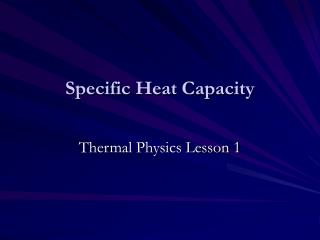DownloadDownload PresentationSpecific Heat Capacity

# Specific Heat Capacity

Download Presentation## Specific Heat Capacity

- - - - - - - - - - - - - - - - - - - - - - - - - - - E N D - - - - - - - - - - - - - - - - - - - - - - - - - - -
##### Presentation Transcript

1. Specific Heat Capacity Thermal Physics Lesson 1

2. Learning Objectives • Define specific heat capacity. • Perform calculations using ∆Q=mc ∆θ. • Describe how specific heat capacity can be measured in the lab.

3. Homework • Complete worksheet to practice calculations we will look at today.

4. Why do we care • The large SHC of water is particularly important for the development and maintenance of life on Earth.

5. Why are we learning this? • Historical Context • Images of Joule, Kelvin • Steam engines • Thermal physics in stars

6. Which is hotter? Bath water or a lit match?Which needed more energy to heat it?

7. Heat vs. Temperature • Heat as water and temperature as wetness analogy • ‘Heat’ is not an entity but a short hand name for a process (heating as oppose to working).

8. State vs. Phase • Solids, liquids and gases are three of the different phases of matter (superfluids and plasmas are two others. Thus melting, boiling etc are changes of phase. • Each phase can exist in a variety of states depending upon e.g. the temperature and pressure. •  However, the exam board uses “state” to mean phase.

9. Specific Heat Capacity • Definition:- • The specific heat capacity (c) of a substance is the amount of energy needed to raise the temperature of 1kg of the substance by 1K (or 1 ºC)

10. Specific Heat Capacity Equation: where:- ∆Q is the energy change in J m is the mass in kg c is the specific heat capacity in J K-1 kg-1 ∆θ is the temperature change in K

11. Worked Example 1 • A bucket containing 11.5 litres of cold water at 10°C is taken into a house at a warmer temperature and left inside until it has reached thermal equilibrium with it new surroundings. • If 504 kJ of energy is absorbed from the surroundings to heat the water, what is the temperature of the room?

12. Worked Example 1 • A bucket containing 11.5 litres of cold water at 10°C is taken into a house at a warmer temperature and left inside until it has reached thermal equilibrium with it new surroundings. • If 504 kJ of energy is absorbed from the surroundings to heat the water, what is the temperature of the room? • ∆Q =504 kJ • m=11.5 kg • c = 4200 J kg-1 °C-1 • ∆θ = ? Using ∆ θ= 504,000 J / (11.5kg × 4200 J K-1°C-1 ) = 10.4 °C (to 3 s.f.) So temperature of the water = 10°C + 10.4 °C = 20.4 °C Temperature of the room = T of water in thermal eqm. = 20.4 °C

13. Worked Example 2 • A 60 W immersion heater takes 2.5 minutes to heat 0.5 kg of water from 21 to 25, what is the specific heat capacity of the water. • P = 60 W • ∆ t = 2.5 minutes = (2.5 × 60)s = 150 s • ∆θ = θ2 -θ1 = 4 oC = 4 K • m=0.5 kg • c =? • ∆Q = P ∆ t = 60 W × 150 s = 9,000 J • c = 9,000 J / (0.5 kg × 4 K) = 4,500 J kg-1 K-1 • Why is it higher than the accepted value of c = 4200 J kg-1 K-1?

14. Note • If a question includes heating and then melting a substance then use:-